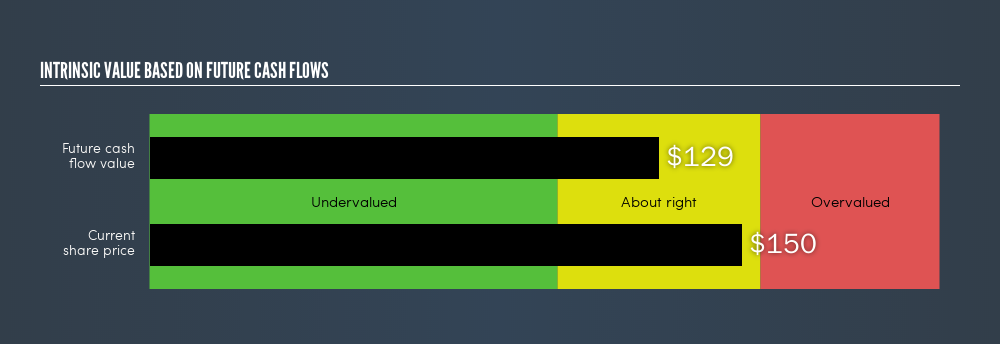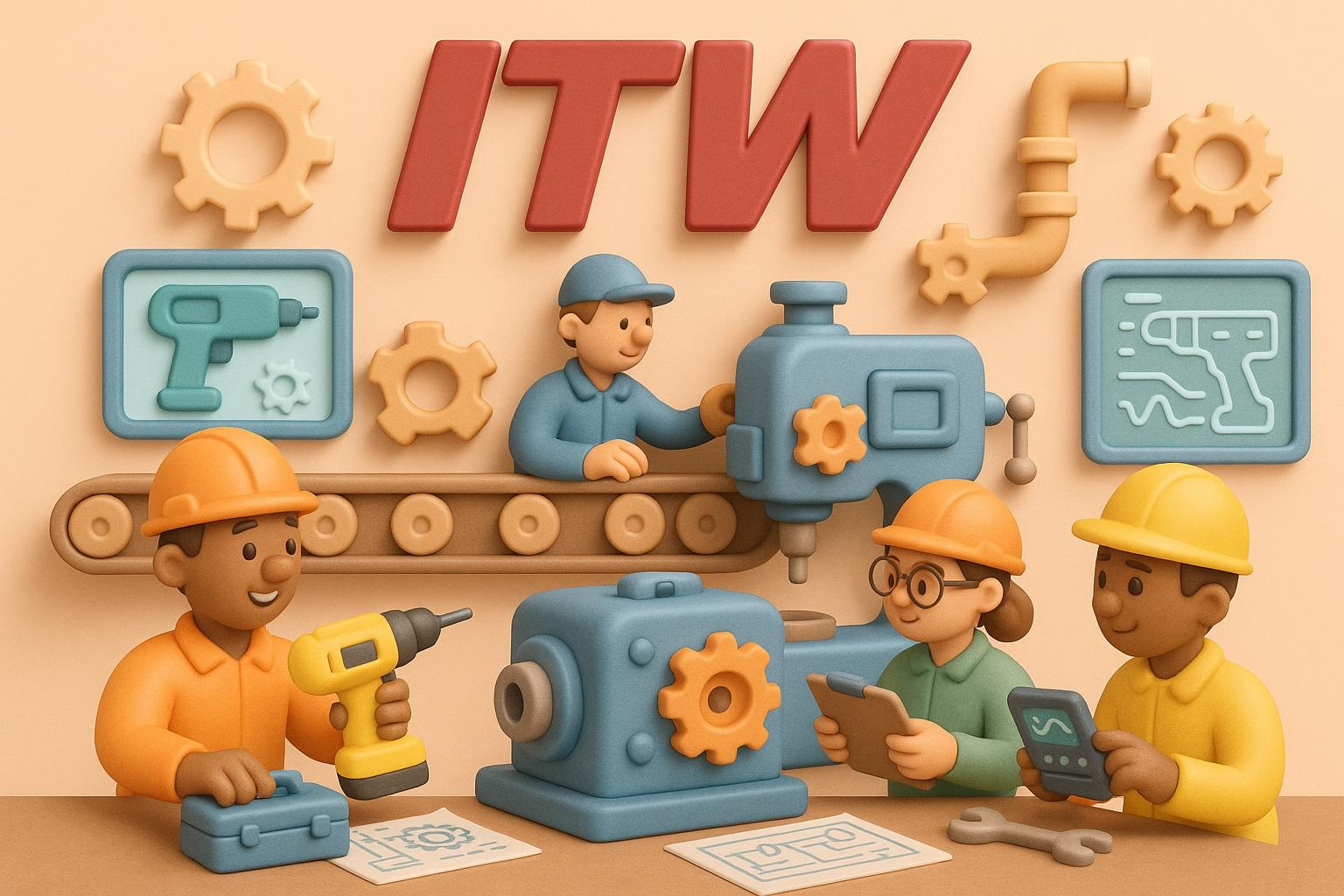latest

# Calculating The Fair Value Of Illinois Tool Works Inc. (NYSE:ITW)

Today we will run through one way of estimating the intrinsic value of Illinois Tool Works Inc. (NYSE:ITW) by taking the expected future cash flows and discounting them to their present value. I will be using the Discounted Cash Flow (DCF) model. Don’t get put off by the jargon, the math behind it is actually quite straightforward.

Remember though, that there are many ways to estimate a company’s value, and a DCF is just one method. Anyone interested in learning a bit more about intrinsic value should have a read of the Simply Wall St analysis model.

### Crunching the numbers

We are going to use a two-stage DCF model, which, as the name states, takes into account two stages of growth. The first stage is generally a higher growth period which levels off heading towards the terminal value, captured in the second ‘steady growth’ period. To start off with, we need to estimate the next ten years of cash flows. Where possible we use analyst estimates, but when these aren’t available we extrapolate the previous free cash flow (FCF) from the last estimate or reported value. We assume companies with shrinking free cash flow will slow their rate of shrinkage, and that companies with growing free cash flow will see their growth rate slow, over this period. We do this to reflect that growth tends to slow more in the early years than it does in later years.

A DCF is all about the idea that a dollar in the future is less valuable than a dollar today, and so the sum of these future cash flows is then discounted to today’s value:

#### 10-year free cash flow (FCF) estimate

 2020 2021 2022 2023 2024 2025 2026 2027 2028 2029 Levered FCF (\$, Millions) \$2.7b \$2.8b \$2.9b \$3.0b \$3.1b \$3.2b \$3.3b \$3.4b \$3.5b \$3.6b Growth Rate Estimate Source Analyst x8 Analyst x5 Analyst x2 Analyst x2 Est @ 3.01% Est @ 2.93% Est @ 2.87% Est @ 2.83% Est @ 2.8% Est @ 2.78% Present Value (\$, Millions) Discounted @ 9.41% \$2.5k \$2.4k \$2.2k \$2.1k \$2.0k \$1.9k \$1.7k \$1.6k \$1.5k \$1.5k

(“Est” = FCF growth rate estimated by Simply Wall St)
Present Value of 10-year Cash Flow (PVCF)= \$19.4b

The second stage is also known as Terminal Value, this is the business’s cash flow after the first stage. For a number of reasons a very conservative growth rate is used that cannot exceed that of a country’s GDP growth. In this case we have used the 10-year government bond rate (2.7%) to estimate future growth. In the same way as with the 10-year ‘growth’ period, we discount future cash flows to today’s value, using a cost of equity of 9.4%.

Terminal Value (TV) = FCF2029 × (1 + g) ÷ (r – g) = US\$3.6b × (1 + 2.7%) ÷ (9.4% – 2.7%) = US\$55b

Present Value of Terminal Value (PVTV) = TV / (1 + r)10 = \$US\$55b ÷ ( 1 + 9.4%)10 = \$22.33b

The total value, or equity value, is then the sum of the present value of the future cash flows, which in this case is \$41.73b. To get the intrinsic value per share, we divide this by the total number of shares outstanding. This results in an intrinsic value estimate of \$129.01. Relative to the current share price of \$150.22, the company appears around fair value at the time of writing. The assumptions in any calculation have a big impact on the valuation, so it is better to view this as a rough estimate, not precise down to the last cent.NYSE:ITW Intrinsic value, August 19th 2019

### The assumptions

We would point out that the most important inputs to a discounted cash flow are the discount rate and of course the actual cash flows. If you don’t agree with these result, have a go at the calculation yourself and play with the assumptions. The DCF also does not consider the possible cyclicality of an industry, or a company’s future capital requirements, so it does not give a full picture of a company’s potential performance. Given that we are looking at Illinois Tool Works as potential shareholders, the cost of equity is used as the discount rate, rather than the cost of capital (or weighted average cost of capital, WACC) which accounts for debt. In this calculation we’ve used 9.4%, which is based on a levered beta of 1.12. Beta is a measure of a stock’s volatility, compared to the market as a whole. We get our beta from the industry average beta of globally comparable companies, with an imposed limit between 0.8 and 2.0, which is a reasonable range for a stable business.

### Next Steps:

Whilst important, DCF calculation shouldn’t be the only metric you look at when researching a company. The DCF model is not a perfect stock valuation tool. Rather it should be seen as a guide to “what assumptions need to be true for this stock to be under/overvalued?” If a company grows at a different rate, or if its cost of equity or risk free rate changes sharply, the output can look very different. For Illinois Tool Works, There are three fundamental factors you should further research:

1. Financial Health: Does ITW have a healthy balance sheet? Take a look at our free balance sheet analysis with six simple checks on key factors like leverage and risk.
2. Future Earnings: How does ITW’s growth rate compare to its peers and the wider market? Dig deeper into the analyst consensus number for the upcoming years by interacting with our free analyst growth expectation chart.
3. Other High Quality Alternatives: Are there other high quality stocks you could be holding instead of ITW? Explore our interactive list of high quality stocks to get an idea of what else is out there you may be missing!

PS. Simply Wall St updates its DCF calculation for every US stock every day, so if you want to find the intrinsic value of any other stock just search here.

We aim to bring you long-term focused research analysis driven by fundamental data. Note that our analysis may not factor in the latest price-sensitive company announcements or qualitative material.

If you spot an error that warrants correction, please contact the editor at editorial-team@simplywallst.com. This article by Simply Wall St is general in nature. It does not constitute a recommendation to buy or sell any stock, and does not take account of your objectives, or your financial situation. Simply Wall St has no position in the stocks mentioned. Thank you for reading.